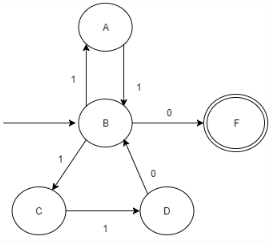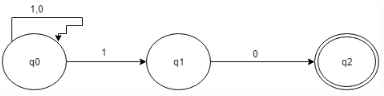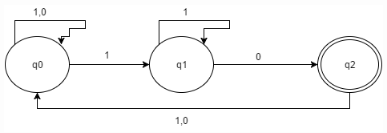Courses

# Test: The Language of NFA

## 10 Questions MCQ Test Theory of Computation | Test: The Language of NFA

Description
This mock test of Test: The Language of NFA for Computer Science Engineering (CSE) helps you for every Computer Science Engineering (CSE) entrance exam. This contains 10 Multiple Choice Questions for Computer Science Engineering (CSE) Test: The Language of NFA (mcq) to study with solutions a complete question bank. The solved questions answers in this Test: The Language of NFA quiz give you a good mix of easy questions and tough questions. Computer Science Engineering (CSE) students definitely take this Test: The Language of NFA exercise for a better result in the exam. You can find other Test: The Language of NFA extra questions, long questions & short questions for Computer Science Engineering (CSE) on EduRev as well by searching above.
QUESTION: 1

### Subset Construction method refers to:

Solution:

The conversion of a non-deterministic automata into a deterministic one is a process we call subset construction or power set construction.

QUESTION: 2

### Given Language: Ln= {xϵ {0,1} * | |x|≥n, nth symbol from the right in x is 1} How many state are required to execute L3 using NFA?

Solution:

The finite automaton for the given language is made and thus, the answer can be obtained.

QUESTION: 3

### Which of the following does the given NFA represent?Solution:

The given diagram can be analysed and thus the option can be seeked.

QUESTION: 4

The number of transitions required to convert the following into equivalents DFA:Solution:QUESTION: 5

If L is a regular language, Lc and Lr both will be:

Solution:

If L is a regular Language, Lc and Lr both are regular even.

QUESTION: 6

In NFA, this very state is like dead-end non final state:

Solution:

REJECT state will be like a halting state which rejects a particular invalid input.

QUESTION: 7

We can represent one language in more one FSMs, true or false?

Solution:

We can represent one language in more one FSMs, example for a same language we have a DFA and an equivalent NFA.

QUESTION: 8

The production of form non-terminal -> ε is called:

Solution:

The production of form non-terminal ->ε is call null production.

QUESTION: 9

Which of the following is a regular language?

Solution:

DFSM’s for the first three option is not possible; hence they aren’t regular.

QUESTION: 10

Which of the following recognizes the same formal language as of DFA and NFA?

Solution:

All the three option refers to same technique if distinguishing similar constructions for different type of automata.